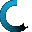Retrieving data ...
Please do not click anywhere else.

If you see no response after 2-3 minutes, then click on the [X] button in the upper right corner to close the window and retry the operation at a later time.
Interactive Multimedia Learning & Teaching Management System Suite## Course Contents

• 1. Counting Numbers
•  1.1 Counting Numbers to 100
•  1.2 Reading and Writing Numbers to 100
•  1.3 Identifying Numbers to 100
•  1.4 Find the Missing Number
•  1.5 Reverse Counting
•  1.6 One More, One Less
•  1.7 Skip Counting by Two
•  1.8 Skip Counting by Five
•  1.9 Name the Number
•  1.10 Identifying Numbers past 100
•  1.11 Skip Counting by Ten
•  1.12 Skip Counting by Twenty-five
• 2. Number Sense
•  2.1 Number Types
•  2.2 Even and Odd Numbers
•  2.3 Introduction to Place Values
•  2.4 Identifying Ones and Tens in Numbers
•  2.5 Number Combinations
•  2.6 The Role of Zero
• 3. Comparing and Ordering Numbers
•  3.1 Comparing 1-Digit Numbers
•  3.2 Comparing 1 and 2-Digit Numbers
•  3.3 Comparing 2-Digit Numbers
•  3.4 Arranging 1-Digit Numbers in Ascending Order
•  3.5 Arranging 1-Digit Numbers in Descending Order
•  3.6 Arranging 1 and 2-Digit Numbers in Ascending Order (Optional)
•  3.7 Arranging 2-Digit Numbers in Ascending Order (Optional)
•  3.8 Arranging 1 and 2-Digit Numbers in Descending Order (Optional)
•  3.9 Arranging 2-Digit Numbers in Descending Order (Optional)
• 4. Addition - Part 1
•  4.5 Find 10 More
•  4.6 Commutative Property of Addition
•  4.7 Associative Property of Addition
•  4.9 True or False
•  4.10 Find the Missing Number
• 5. Addition - Part 2
•  5.1 Addition without Carrying Numbers (Optional)
•  5.2 Addition with Carrying Numbers
•  5.3 Adding a 2-digit Number and a 1-digit Number
•  5.4 Adding a 2-digit Number and a 2-digit Number
•  5.5 Adding 3 Number Sets
• 6. Subtraction - Part 1
•  6.1 Introduction to Subtraction
•  6.2 Subtraction Using Objects
•  6.3 Identity Property of Subtraction
•  6.4 Subtraction in Relation to Addition
•  6.5 Subtraction Using a Subtraction Table
•  6.6 Subtraction Using Regrouping
•  6.7 Find 10 Less
•  6.8 True or False
•  6.9 Find the Missing Number
• 7. Subtraction - Part 2
•  7.1 Subtraction without Borrowing Numbers (Optional)
•  7.2 Subtraction with Borrowing Numbers
•  7.3 Subtracting a 1-digit Number from a 2-digit Number
•  7.4 Subtracting a 2-digit Number from a 2-digit Number
• 8. Fractions
•  8.1 Introduction to Fractions
•  8.2 Identifying Fractions
•  8.3 Writing Fractions
•  8.4 Fractions Using Objects
•  8.5 Equivalent Fractions
• 9. Graphs
•  9.1 Introduction to Picture and Bar Graphs
•  9.2 Creating Picture Graphs
•  9.3 Interpreting Picture Graphs
•  9.4 Creating Bar Graphs
•  9.5 Interpreting Bar Graphs
• 10. Time
•  10.1 Days, Weeks, Months and Years
•  10.2 Days
•  10.3 Weeks
•  10.4 Months
•  10.5 Years
•  10.6 The Four Seasons
•  10.7 Seconds, Minutes and Hours
•  10.8 Digital and Analog Clocks
•  10.9 Telling Time to the Hour
•  10.10 Telling Time to the Half-hour
•  10.11 Telling Time to the Quarter-hour
• 11. Money
•  11.1 Pennies, Nickels, Dimes, Quarters
•  11.2 Converting a Nickel, Dime and Quarter to Pennies
•  11.3 Counting Coins up to One Dollar
•  11.4 Comparing Money
• 12. Measurements
•  12.1 Systems of Measurement
•  12.2 Length
•  12.3 Using Non-standard Units to Measure Length
•  12.4 Using Standard Units to Measure Length
•  12.5 Comparing Length
•  12.6 Weight
•  12.7 Temperature
• 13. Geometry
•  13.1 Introduction to Geometry
•  13.2 Naming Plane and Solid Shapes
•  13.3 Dimensions of Shapes
•  13.4 Vertices, Edges and Faces
•  13.5 Polygons
•  13.6 Identifying Plane and Solid shapes
•  13.7 Working with Shapes
• 14. Algebra
•  14.1 Recognizing and Predicting Patterns
•  14.2 Extending Patterns
•  14.3 Increasing Number Patterns
•  14.4 Decreasing Number Patterns
•  14.5 Balancing Equations
•  14.6 Find the Missing Number